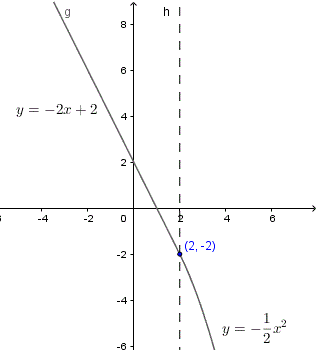# Questions on Continuity with Solutions

Questions with answers on the continuity of functions with emphasis on rational and piecewise functions. The continuity of a function and its derivative at a given point is discussed. Graphical meaning and interpretation of continuity are also included.

Example 1: For what values of x are each of the following functions discontinuous?

a) \;\; f(x) = \dfrac{1}{x} \;\; b) \;\; g(x) = \dfrac{2}{x-2} \;\; c) \;\; h(x) = \dfrac{x+1}{x^2-1}

d) \;\; i(x) = \tan(x) \;\; e) \;\; j(x) = \dfrac{1}{\cos(x)-1} \;\; f) \;\; k(x) = \dfrac{x+2}{x^2+5}

g) \;\; l(x) = \begin{cases} \dfrac{x+4}{x+4} & x \ne -4 \\ 1 & x = -4 \\ \end{cases}

Solution to Example 1
a) For x = 0, the denominator of function f(x) is equal to 0 and f(x) is not defined and does not have a limit at x = 0. Therefore function f(x) is discontinuous at x = 0.
b) For x = 2 the denominator of function g(x) is equal to 0 and function g(x) not defined at x = 2 and it has no limit. Function g(x) is not continuous at x = 2.
c) The denominator of function h(x) can be factored as follows: x2 -1 = (x - 1)(x + 1). The denominator is equal to 0 for x = 1 and x = -1 values for which the function is undefined and has no limits. Function h is discontinuous at x = 1 and x = -1.
d) tan(x) is undefined for all values of x such that x = ?/2 + k ? , where k is any integer (k = 0, -1, 1, -2, 2,...) and is therefore discontinuous for these same values of x.
e) The denominator of function j(x) is equal to 0 for x such that cos(x) - 1 = 0 or x = k (2 ?), where k is any integer and therefore this function is undefined and therefore discontinuous for all these same values of x.
f) Function k(x) is defined as the ratio of two continuous functions (with denominator x2 + 5 never equal to 0), is defined for all real values of x and therefore has no point of discontinuity.
g) l(x) = (x + 4)/(x + 4) = 1 . Hence lim l(x) as x approaches -4 = 1 = l(-4) . Function l(x) is continuous for all real values of x and therefore has no point of discontinuity.

Example 2: Find b such that f(x) given below is continuous?

f(x) = \begin{cases} 2x^2+b & x \ge -1 \\ -x^3 & x \textless -1 \\ \end{cases}

Solution to Example 2
For x > -1, f(x) = 2 x 2 + b is a polynomial function and therefore continuous.
For x < -1, f(x)= -x 3 is a polynomial function and therefore continuous.
For x = -1
f(-1) = 2(-1) 2 + b = 2 + b
let us consider the left and right hand limits
limit from left of -1

L1 = \lim_{x\to\ -1^-} f(x) = -(-1)^3 = 1

limit from right of -1
L2 = \lim_{x\to\ -1^+} f(x) = 2(-1)^2 + b = 2 + b

For function f to be continuous, we need to have
L1 = L2 = 2 + b
or 2 + b = 1 or b = -1.
Substitute b by -1 in the given function to obtain
f(x) = \begin{cases} 2x^2-1 & x \ge -1 \\ -x^3 & x \textless -1 \\ \end{cases}
The graph of f is shown below and it is clear that the function is continuous at x = -1.Example 3: Find a and b such that both g(x) given below and its first derivative are continuous?

g(x) = \begin{cases} ax^2+b & x \ge 2 \\ -2x+2 & x \textless 2 \\ \end{cases}

Solution to Example 3
Continuity of function g
For x > 2, g(x) = a x 2 + b is a polynomial function and therefore continuous.
For x < 2, g(x) = -2 x + 2 is a polynomial function and therefore continuous.
let

L1 = \lim_{x\to\ 2^+} g(x) = a (2)^2 + b = 4 a + b

L2 = \lim_{x\to\ 2^-} g(x) = -2(2) + 2 = -2

For continuity of g at x = 2, we need to have
L1 = L2 = g(2)
Which gives
4 a + b = -2
Continuity of the derivative g'
For x > 2, g '(x) = 2 a x is a polynomial function and therefore continuous.
For x < 2, g '(x) = -2 is a constant function and therefore continuous.
Let
l1 = \lim_{x\to\ 2^+} g'(x) = 2a(2) = 4 a

l2 = \lim_{x\to\ 2^-} g'(x) = -2

For continuity of g' at x = 2, we need to have
l1 = l2 or 4 a = - 2
The last equation gives: a = - 1 / 2. And substitute a by - 1 / 2 in the equation 4 a + b = -2 obtained above, we obtain b = 0. Substitute a and b by their values to obtain:
g(x) = \begin{cases} -\dfrac{1}{2}x^2 & x \ge 2 \\ -2x+2 & x \textless 2 \\ \end{cases}
Function g(x) is graphed below and it is clear that both the function and its derivative (slope) are continuous at x = 2.More on Continuous Functions in Calculus
and Continuity Theorems and Their use in Calculus